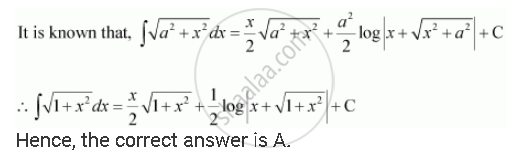# Choose the Correct Answer Int Sqrt(1+ X^2) Dx is Equal - Mathematics

#### Question

Choose the correct answer int sqrt(1+ x^2) dx is equal

(A) x/2 sqrt(1+ x^2) +  1/2log |(x + sqrt(1+ x^2)) + C|

(B) 2/3 (1+ x^2)^(3/2) + C

(C) 2/3x(1 + x^2)^(3/2) + C

(D) x^2/2 sqrt(1+x^2) + 1/2 x^2 log|x + sqrt(1+ x^2)|+ C

#### Solution 1#### Solution 2Is there an error in this question or solution?

#### Video TutorialsVIEW ALL 

Choose the Correct Answer Int Sqrt(1+ X^2) Dx is Equal Concept: Integrals of Some Particular Functions.